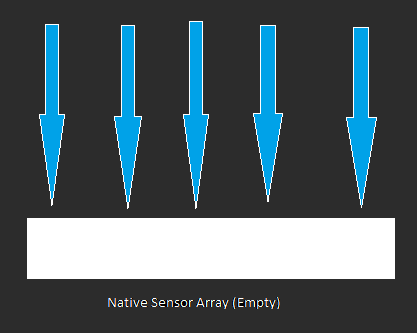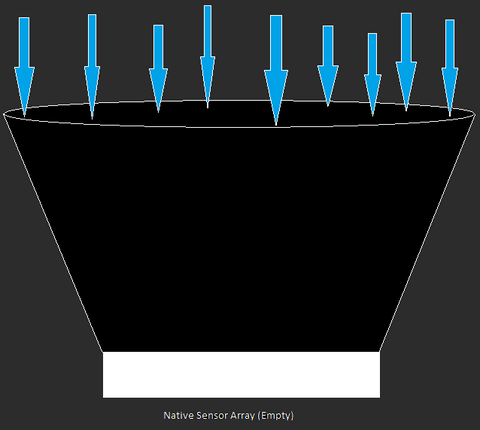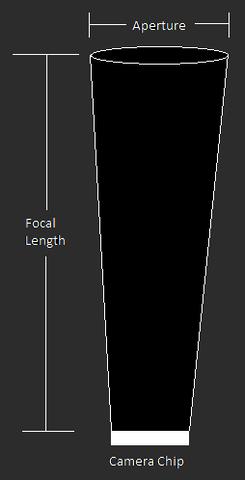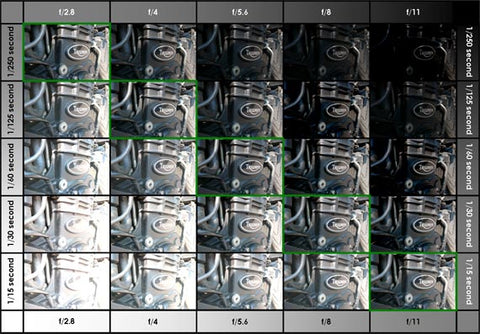# Astrophotography, Pixel by Pixel: Part 2 - Focal Ratio Effects

## Astrophotography, Pixel by Pixel: Part 2

Welcome to part 2 of this blog series, “Astrophotography, Pixel-by-Pixel.” For a quick review of where we left off, you can find part 1 of the series here. It would be useful to review the concepts covered, such as well-depth, quantum efficiency, and the effects of different pixel sizes. We will be building upon those basics, and jump into some further details and nuances of different imaging systems. So grab your buckets, and let’s jump on down back to a single pixel!

## FOCAL RATIO EFFECTS

For visual astronomy, it is absolutely the case that aperture is king. The larger the aperture of your telescope, the more light-gathering power it has, and the finer detail it can resolve. While aperture cannot be completely ignored in astrophotography, often what we care about more is the focal ratio of the telescope. The focal ratio is a unitless number, and can be found by dividing the focal length of the telescope by the aperture.

For example, if a telescope had 80mm of aperture and a focal length of 500mm, then the focal ratio of the scope would be 500mm/80mm = f/6.25. We can see the effects on this change if we alter one or the other of these measurements at a time. Say that we keep the 80mm aperture the same but increase the focal length to 800mm. Our focal ratio then becomes f/10. If we keep the focal length constant at 500mm but increase the aperture to 125mm, then the focal ratio then becomes f/4.

Well that is a decent bit of math for our buckets! Let’s apply this idea back to our analogy. With our buckets out on the lawn collecting rain, they just collect however much rainwater would fall on their exact position. But what if we could increase the rate at which our buckets collected the water? That is exactly what a telescope does. Recall that in this analogy, each bucket is a single pixel, and our array of all the buckets is the entire camera sensor, and incoming rain water is the light coming in from our astronomical object we are imaging. A telescope acts like a big funnel: it gathers more light over a given amount of time to put on the sensor.(Figure 1: Camera Chip Without Scope)(Figure 2: Camera Chip With Telescope or Lens)

If aperture is king for visual astronomy, then why is it the case that in astrophotography we care more about the f/ratio? The shortest answer as to why we care about focal ratio so much is that f/ratio is what determines how much light falls onto each of our pixels. We definitely want to maximize the amount of light gathered by each pixel since the objects we are imaging are so very comparatively dim, and the lower the f/ratio, the more light hits each pixel. Let’s shrink down our sensor from before and see what happens when we change one part of our imaging train at a time that affects the focal ratio.

Take the following diagram as the starting point. As we can see, we are still gathering more light (rain) on our sensor (all of our buckets in an array) than without a scope at all. Now let’s change some of the measurements on this starting point to see their effects.(Figure 3: Focal Ratio Baseline)

We’ll begin by keeping the same focal length, but increasing the aperture of the telescope. This would result in a lower f/ratio (recall that the f/ratio is focal length divided by aperture), or a system that gathers more light for the sensor over the same amount of time. Each pixel-bucket is getting more light as compared to the base example.(Figure 4: Larger Aperture, Lower Focal Ratio)

It should be clear that moving in the other direction (keeping the focal length the same, but decreasing the aperture) results in a higher f/ratio, which is a slower system because there is less light hitting each pixel in the same amount of time.

So how about changing the other measurement that is tied to focal ratio? Let’s look at what happens when we keep the aperture the same but increase the focal length. This results in a higher f/ratio and a slower system. It may be a little less intuitive to see, but by keeping the opening the same but basically increasing the distance, we are making the system become closer to vertical. A perfectly “vertical” system would be the exact same as having no telescope helping you gather light on your sensor.(Figure 5: Longer Focal Length, Higher Focal Ratio)

The takeaway from all of this is that we want to have a fast scope for astrophotography because it allows us to gather light more quickly. This lets us either get more light for the same duration of shot, or we can lower our exposure duration to gather the equivalent amount of light as a slower focal ratio system which eases the burden on our imaging system.https://i.pinimg.com/originals/d9/ef/d5/d9efd58c8753c9d7d100c629bc8ae8a0.jpg

(Figure 6: Focal Ratio and Exposure Duration)

Each full f/stop change helps (or harms) your signal to noise ratio by 41%. This is ultimately why we care about focal ratio so much in astrophotography. I would much rather be under-sampled with a low focal ratio system. When looking to optimize your imaging system, go for as low of an f/ratio as you can get!

There is one further aspect to consider here with this 41% increase and focal ratio effects: all of the above assumed a straight through optical path like you would find in refractor telescopes. So I ask an open question: what do you think would happen if there were a central obstruction such as on a Schmidt-Cassegrain or a Newtonian telescope? We'd love to hear your thoughts in the comments section.

## Takeaways

1) Focal ratio (f/ratio) matters more than aperture for astrophotography (though aperture cannot be completely ignored)

2) Aim for a low focal ratio gear setup

You can continue reading the next part of this series here.

• You are a very good teacher :) Thank you!

Tim
• Easy to understand, im not able to understand most explanations on this topic. To many mathematical equations. I got into this for pleasure not headaches. thanks for being normal, and saving me another headache!

Mike
• Clear and easy explanation. thanks a lot.

Arthur Safar
• Just AMAZINGLY well written article… so helpful, thank you bery much!

Marco Solano